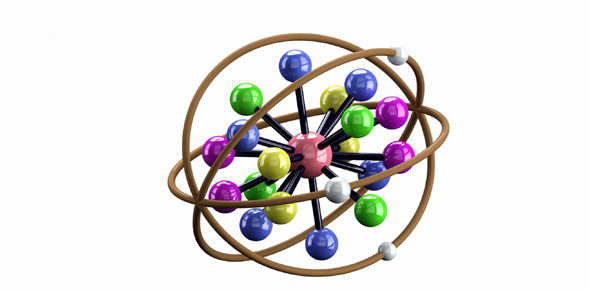# Ionic Bonding Quiz Grade 8a

13 Questions | Attempts: 893SettingsIon formation, valence and ionic bonding review.

• 1.
Ionic bonds always form between metals and ___________.
• A.

Nonmetals

• B.

Metals

• C.

Metalloids

• 2.
Negatively charged ions, called ____________, result from gain of electrons.
• A.

Cations

• B.

Anions

• 3.
Positively charged ions, called ____________, result from loss of electrons.
• A.

Cations

• B.

Anions

• 4.
When an electron is added to or removed from an atom, the atom becomes a(n) ________.
• A.

Ion

• B.

Bond

• C.

Nothing; it stays an atom

• 5.
How does a magnesium atom form a bond with an oxygen atom?
• A.

By giving one pair of electrons to the oxygen atom.

• B.

By sharing one pair of electrons, both electrons provided by the magnesium atom.

• C.

By sharing two pairs of electrons, both pairs provided by the oxygen atom.

• D.

By sharing two pairs of electrons, each atom donating one pair of electrons.

• 6.
Lewis dot shows?
• A.

The total number of electrons

• B.

Total number of protons

• C.

Total number of neutrons

• D.

Number of electrons in highest energy shelf

• 7.
Select the formula for strontium chloride.
• A.

SrCl2

• B.

SrCl

• C.

Sr2Cl3

• D.

SrClO2

• E.

SrClO3

• 8.
Select the formula for aluminum sulfide.
• A.

Al2(SO4)3

• B.

AlS

• C.

Al2S3

• D.

Al3(SO3)2

• E.

AlSO3

• 9.
Element X has an electronic structure 2, 8, 8, 1. Element Y has an electronic structure of 2, 8, 6. What is the chemical formula of the compound formed between X and Y?
• A.

X2Y

• B.

XY2

• C.

XY

• D.

XY3

• 10.
Which element, when combined with fluorine, would most likely form an ionic compound?
• A.

Phosphorus

• B.

Lithium

• C.

Carbon

• D.

Chlorine

• 11.
Aluminum is a group 3 metal. Which ion does Al typically form?
• A.

Al3-

• B.

Al5+

• C.

Al3+

• D.

Al5-

• 12.
NaCl is an example of the chemical formula of
• A.

An ion

• B.

A covalent compound

• C.

An ionic compound

• D.

A crystal

• 13.
Which pair of elements will combine to form an ionic compound?
• A.

Carbon and chlorine

• B.

Fluorine and sodium

• C.

Sulphur and oxygen

• D.

Oxygen and carbon

## Related TopicsBack to top
×

Wait!
Here's an interesting quiz for you.